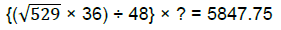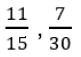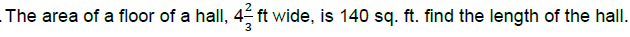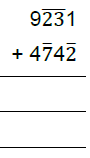Welcome to your Vedic Math Sr. Paper-II

Name
1.
Find the value of x and y by Vedic Method:

a)  2x + 3y = 13
b) 5x + 2y = 16

2.
Multiply the following by verticle and cross wise method:

(7x – 3 ) and (3x – 1)

3.
Which is the right option:4.
FInd the value of 92 × 98

5.
Digit sum of a two digit number is 6. First digit is double the 2nd digit. Find the number

6.
11121 ÷ 9, Find the quotient and remainder

7.
Divide 37 by 25 ( by Vedic method)

8.
Without actual solving find which of the following will be correct answer:

21821 X 7894

9.
Find the Cube Root of 153130375

10.
Solve the following quadratic Equation by vedic method:

2x^2 -11x +15

11.
Which one of the following is smaller:12.13.
The ratio of the area of two classroom floors is 4:5. If the smaller classroom has a floor of 30 Sq m. Find the area of larger classroom.

14.
Find the square Root of 451584

15.
What will be day on 26th Jan 2051

16.
Rishi sold his television set for Rs 2560, thereby losing 20% on what he paid for it. What did he pay for it?

17.